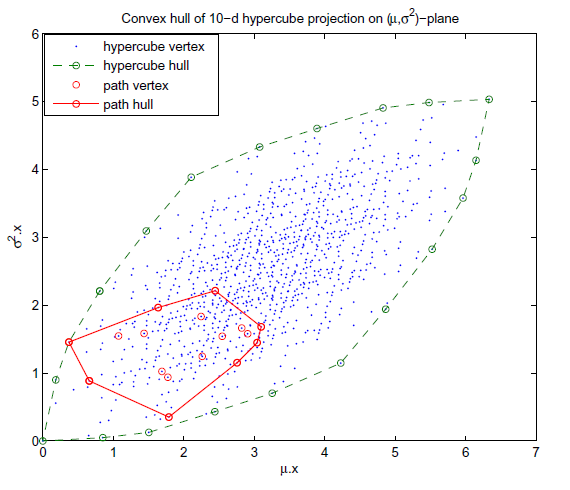Stochastic Shortest Paths Via Quasi-convex Maximization

•  Nikolova, E.; Kelner, J.; Brand, M.; Mitzenmacher, M., "Stochastic Shortest Paths Via Quasi-convex Maximization", European Symposium on Algorithms (ESA), ISBN: 3-540-38875-3, September 2006, pp. 552-563.
• @inproceedings{Nikolova2006sep,
• author = {Nikolova, E. and Kelner, J. and Brand, M. and Mitzenmacher, M.},
• title = {Stochastic Shortest Paths Via Quasi-convex Maximization},
• booktitle = {European Symposium on Algorithms (ESA)},
• year = 2006,
• pages = {552--563},
• month = sep,
• isbn = {3-540-38875-3},
• url = {https://www.merl.com/publications/TR2006-128}
• }
• Research Area:Projection of the unit hypercube (representing all edge subsets) and the path polytope onto the plane.

We consider the problem of finding shortest paths in a graph with independent randomly distributed edge lengths. Our goal is to maximize the probability that the path length does not exceed a given threshold value (deadline). We give a surprising exact n theta (log n) algorithm for the case of normally distributed edge lengths, which is based on quasi-convex maximization. We then prove average and smoothed polynomial bounds for this algorithm, which also translate to average and smoothed bounds for the parametric shortest path problem, and extend to a more general non-convex optimization setting. We also consider a number other edge length distributions, giving a range of exact and approximation schemes.